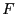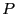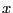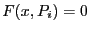Next: Choosing the right heuristics Up: Large computation time Previous: Large computation time   Contents

Changing the formulation of the problem

Interval analysis is a very specific domain: as a naive user you may want to solve a specific problem but the problem that you have submitted to ALIAS may be already a transformed version of the problem you want really to solve and this transformation may be not favorable to the use of interval analysis. Focusing on the problem you want to solve is important for the use of interval analysis.

Rule: focus on the problem you want to solve

Let us give you an example: you have a third order polynomialdepending on a set of parametersand you want to find if there are values of these parameters such that the rootof the polynomial verifies some properties. A natural way of doing that is to use the available generic analytical form of the root and check if you may find parameters such thatsatisfy the properties. In terms of interval analysis you have already transformed your problem and this may not be a good idea (the analytical form of the roots of a 3rd order polynomial is badly conditioned).

Rule: it is usually a bad idea to try to adapt to interval analysis the last step of a solving problem that has already been transformed to fit another method

Thinking backward what you want really is to verify if thesatisfyingsatisfy the properties. Hence you should use as unknown not only thebut also the: you add an unknown but the calculation will then involve only the evaluation of the polynomial which is much more simple than the evaluation of the analytical form of the roots.

Rule: introducing new variables for simplifying expressions may be a good strategy

The rule for the right formulation is to find the right compromise between the complexity of the expressions (very complex expressions will usually lead to a large overestimation of the expressions) and the number of variables.

A good strategy is also to learn what are the main problems that have been addressed in interval analysis so that you can later on transform your problem into another one for which solving algorithms are available.

Rule: investigate which main problems have been addressed in interval analysis so that you may later on transform your problem into another one for which solving algorithms are available

Typical of such approach is to determine if a parametric matrix may include singular matrices. You may be tempted to derive the determinant of the interval matrix and then to determine if there are values of the unknowns that cancel it. But if you have not been able to manually determine these values this probably means that the determinant is quite complicated, and therefore not appropriate for interval analysis. But determining if a parametric matrix may include a singular matrix is a well-known problem in interval analysis and for solving this problem there are efficient methods that do not require the calculation of the determinant (see section 7.4).

Another case of failure occurs when the system is badly conditioned. This may occur, for example, when you have large coefficients in an equation while the values of the variable are very small: as ALIAS takes into account the round-off errors you will get large interval evaluation even for a small width of the intervals in the input interval (see for example the evaluation of the Wilkinson polynomial in section 5.9.3.2). There is no standard way to solve the problem. The first thing you may try is to normalize the unknowns in order to reduce the size of the coefficients. A second approach may be to switch a solving problem to an optimization problem.

Another trick for system solving is to combine the initial equations to get new one. For example consider the system:

cons1 := a1 + a2 + a3 + 1 ;
cons2 := a1 + 2*a2*x1 + 3*a3*x1^2 + 4*x1^3 ;
cons3 := a1 + 2*a2*x2 + 3*a3*x2^2 + 4*x2^3 ;
cons4 := a1 + 2*a2*x3 + 3*a3*x3^2 + 4*x3^3 ;
cons5 := a1*(x1+x2) + a2*(x1^2 + x2^2) + a3*(x1^3 + x2^3) + x1^4 + x2^4 ;
cons6 := a1*(x2+x3) + a2*(x2^2 + x3^2) + a3*(x2^3 + x3^3) + x2^4 + x3^4 ;
cons7 := x1 + delta -x2<= 0;
cons8 := x2 + delta -x3<= 0;
(which is the fredtest example of our bench). As it this system is difficult to solve (the computation time is over 10 minutes with a 3B increment of 0.001). Now we may notice that monomials involving a1 appear in many equations. Hence we consider adding as equations cons2-cons1, cons2-cons3 and so on. With this additional equations the computation time will be about 1mn 20 seconds.

Rule:Very often introducing additional constraints derived from the set of initial ones will reduce the solving time

Interval arithmetics plays evidently a large role in the computation time. The interval evaluation of mathematical operator such as sine, cosine,...is relatively expensive. If similar complex expressions appear more than once in an expression it is usually very efficient to compute it only once and to substitute it in the other places.

Rule:try to avoid multiple interval evaluation of the same complex expressionsNext: Choosing the right heuristics Up: Large computation time Previous: Large computation time   Contents
Jean-Pierre Merlet 2012-12-20